College Physics : Laws of Thermodynamics and General Concepts

Example Questions

Example Question #1 : Laws Of Thermodynamics And General Concepts

A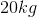steel sword is cooled from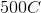to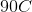by dipping it inof water that is at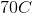If the specific heat of  the steel is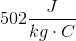and the specific heat of water is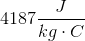, what will be the temperature of the water after the steel is cooled?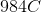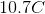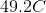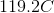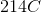Explanation:

This is a simple pairing of two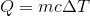equations.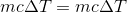We will make the left side for the steel and the right side for water. Thus: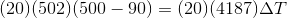Solving for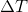of water yields, and certainly the water gained heat not lost it, so the final temperature of the water is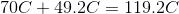Example Question #2 : Laws Of Thermodynamics And General Concepts

A steel beam is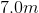long at a temperature of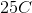. On a hot day, the temperature reaches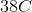. What is the change in the beam's length due to thermal expansion given that the thermal expansion coefficient for steel is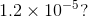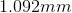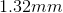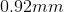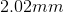Explanation:

We need to use the equation for thermal expansion in order to solve this problem: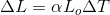We are given: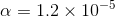for the thermal expansion constant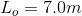for the initial length of the steel.

We need to calculatewhich is the the difference of the two temperatures.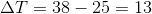Now we have enough information to solve for the change in the length of steel.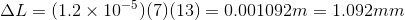Example Question #3 : Laws Of Thermodynamics And General Concepts

What is the change in entropy for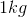of ice initially at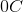that melts to water and warms to the temperature of the atmosphere at?

Latent heat of fusion for water: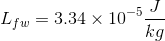Specific heat of water: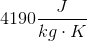Specific heat of ice: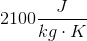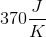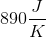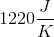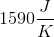Explanation:
• We must account for two separate processes since the ice is melting and then warming. The basic formula to calculate the change in entropy at a constant temperature when the ice melts is:Where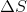is the change in entropy,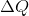is the change in heat energy, and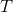is the temperature at which the change of state for the ice occurs. Now we substitute in an equation forfor when ice melts: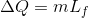. Our entropy equation now becomes: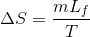Now we plug in our known values into the equation:

Mass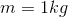Latent heat of fusion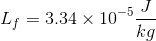Temperature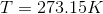And we get the change in entropy for the ice melting at a constant temperature, which is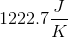• Now we need to calculate the change in entropy for the liquid water warming fromto.

The basic formula to calculate the change in entropy at a changing temperature when the water warms is: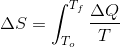Next we substitute in an equation forwhen there is a temperature change, but not a change in state. That equation is: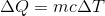so our entropy equation now becomes: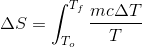next we integrate this equation to get: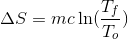Next we plug in the values we know and solve for the change in entropy:

Final temperature of water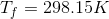Initial temperature of water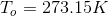Specific heat of water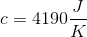Mass of waterNow we get the change in entropy for the water warming to be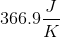We add our two entropy values together to find the total change in entropy to be: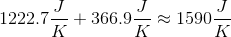All College Physics Resources